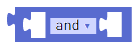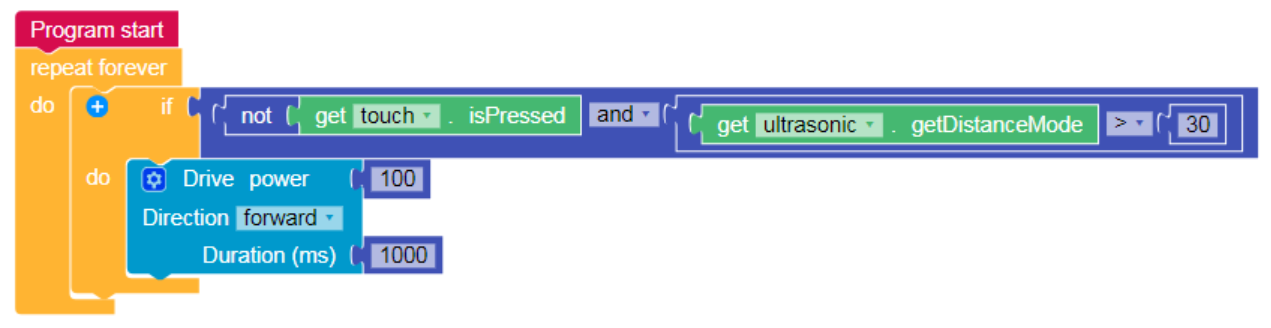# Logical Operator Block

"Logic will get you from A to B. Imagination will take you everywhere." Albert EinsteinThe Logical Operator block returns a Boolean value (True/False) based on the result of two (or more) expressions.

For example, using the Logical Operator block, the following program checks two expressions - that the Touch sensor is not pressed and that the Ultrasonic sensor is far from an obstacle. If both those expressions are true, the robot drives forward.There are four configurations for the Logical Operator block that can be selected using its drop-down menu:

 Operator Description and Returns a true value if both operands (expressions) are true. or Returns a true value if at least one of the operands (expressions) are true. not Returns a true value if the operand (expression) to the right is false, or returns a false value if the operand to the right is  true. xor Returns a true value if only one of the operands (expressions) are true, or returns a false value if both operands are either true or false.